We use cookies to provide our services and for analytics and marketing. You can find out more about our use of cookies here. By continuing to browse our website, you agree to our use of cookies.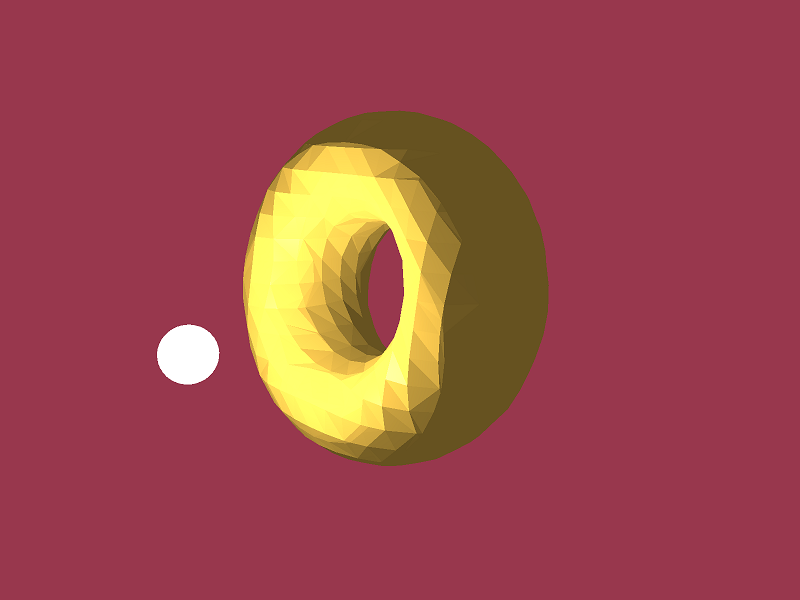# Marching Cubes with OpenGL

## Rendering volumes with C++.

09 November 2020 - D.K.E. Green

Category: Game Design & Development

## Teaser

This post will show how to produce renders like those just below with the marching cubes method using C++ and OpenGL (including GLSL shaders). You can get the source code here.

A sphere with two tunnels.

An implicitly defined torus.

The renders in this article were made with OpenGL (via GLFW). You don't need to follow along with the source, but you can if you like. If you want to get the program running, see README.txt from the source code. You will need CMake and a C++ compiler. Everything should be cross platform, but you may need to include some specific compile definitions on platforms other than Windows. I used Visual Studio 2019 to get everything working, but use whatever build environment makes you happy.

This post will assume some familiarity with C++ and OpenGL, but hopefully you can follow along with the code. The code users shaders, camera transforms and other bits and pieces of math without much explanation.

If you are new to OpenGL, there is a very good and detailed guide here. It is a few years old now, but still worth a read.

If you want more details about this post or get stuck with something, see the contact details or (even better) message me on discord.

## Marching forwards

Online you can find many renders of pixel art worlds. It looks great and I love the 2D aesthetic, but it can make game worlds feel flat.

I like to go on walks to get away from the computer and do some thinking. It had been raining (typical England) most of the night before I set out. My head was off in the clouds, thinking about this bit of code and that, when the universe decided it was time to get back to planet earth. I squelched into a muddy part of the path hidden under some leaves and sank back to reality.

This got me thinking about volumetric modelling of terrain. Standard heightmaps convert an image (with values representing heights) into a single polygonal surface. There is no real depth, heightmap-based terrain provides a visualisation but not a simulation of depth.A 3D rendering produced from a 2D height map. The terrain looks like it has depth, but is only a surface layer. Image courtesy of wikipedia.

To model terrain with actual depth (like the type you could sink into), you need more data. You need to model 'volume' of terrain across a 3D space.

I decided I would put together a tutorial as an excuse to look at a world with some depth.

I didn't want to use any special engine or frameworks, since this would be a side project. I decided to get back to basics and directly use raw OpenGL (with GLSL shaders) and C++.

## What are Marching Cubes?

The original marching cubes paper is here. Advances in this area include dual contouring and the transvoxel method. I will focus on basic marching cubes only.

To model terrain with actual depth (like the type you could sink into), you need to model terrain volumes. No Man's Sky, Astroneer and Minecraft all model the presence or absence of terrain volume through space. Minecraft just directly renders voxels as cubes. Marching cubes is a way of rendering volumetric data more smoothly than something like Minecraft.Marching cube-like terrain in Astroneer.

These algorithms are also used for serious simulations, like fluid flow and soil density models. Marching cubes have also been used to render MRI data. Perhaps details on 'serious' applications of marching cubes would make a good article, but that is for another time.

Marching cubes are easiest to understand in two dimensions (where the technique is often referred to as marching squares). There is an excellent rundown here.

I will give a brief description in words, but the figure just below illustrates the method. I find pictures are more useful for understanding this technique than a written description.

The basic idea behind the rendering technique is to convert a field of scalars over some spatial domain into a set of lines (in 2D) or faces (in 3D). Then, the surface (either lines or faces) computed by the algorithm can be drawn. The drawn surface will represent a particular contour level of the spatial field.

Given a spatial field, an 'iso level' (or contour level) is chosen. Each point in the scalar field needs to be assigned a value in a separate binary spatial field, either 0 or 1. Each point in the spatial field is compared to the iso level. If the scalar field value is less than the iso level, then the binary field is set to 0. If the value in the original scalar field is greater than the iso level, the binary field value is set to 1.

Then, for each cell (in 2D a cell is a square or rectangle with 4 corner points) in the binary field, the render surface is computed. By considering whether or not each cell corner is set to 0 or 1, the cell is assigned a line (in 2D) or face (in 3D) that can be drawn. The drawn lines (or faces) will give a representation of the surface of the iso level.

The marching cubes/squares method defines a particular set of lines/faces. To smooth the drawn surface, interpolation between the values in the original scalar field can be used.Overview of the marching squares method. Image courtesy of wikipedia.

In 3D, the technique is basically the same, but the number of binary field cell cases is much higher. Setting up a program to look up what polygons to draw given the binary field cell corners is a real pain. Luckily, there is code available here which will do the heavy lifting.The original 3D marching cube polygon configurations. Image courtesy of wikipedia.

In this article, I will show how I used this code to draw marching cubes in 3D using OpenGL.

## How to get the marching cubes on the screen?

Note: If you want to follow along with the source code, now is the time to open it up. Follow the instructions in README.txt to get everything working. If you just want to read the article, that's fine too.

I wanted to put this article together using an absolutely minimal GLFW setup. I was trying to build one-off tutorial code and not use any larger framework (which meant not using my own engine - I will write about it one day). That would mean keeping everything as simple as possible.

I had the code from here to compute the polygonisation (the polys required to draw the 3D surface) of each grid cell. Great, but I would still have to set up the grid cell data to call this code.

My plan was to set up reading in a scalar field from a file, then compute the marching cube render surface and render the resultant polygons. I wanted the camera to rotate around the end result, with some basic lighting (just Phong shading).

The scalar field would be defined as a 3D array of numbers from 0 to 1. I could then choose a threshold, the isoLevel, that would define whether or not a vertex should be 'filled' or 'empty' at each position in the binary field 3D array. I would refer to this spatial arrangement as a lattice.

As this was a simple project, I wouldn't bother with a proper fixed time step main loop. It would be enough to just use a locked rendering loop. If I ever need to modify the code, it would be easy to add in a 'proper' loop anyway.

### Shifting the data around

I needed a strategy to get the data on the GPU so I could draw it.

Given a scalar field, the goal is to produce the coordinates of the polygons defining the marching cubes so that they can be drawn:

scalar field --> threshold field --> vertex data


What would be the best approach? There are a few options. Geometry shaders would be great but they are too slow so I virtually never use them. The performance is just really unacceptable, but I understand why. The GPU pipelines are just not set up to handle it well.

The geometry shader option was out.

Another option would be to statically compute the vertex data on startup. I could scan the scalar field sequentially (this could be easily parallelised later). Then, for each 'cell' in the scalar field I could use a lookup table to compute what vertices and edges would need to be added to my mesh. All of this data could be buffered on to the GPU once, so rendering performance would be very fast.

This would be the easiest, but would not allow for dynamic changes to the drawn marching cubes.

A more complex approach based on some GPU buffering and caching tricks would allow for fast, dynamic rendering. That would be great, but really wasn't necessary for this case. I would keep it simple this time. The dynamic method might be a future post.

Static rendering it is. I would still need to write appropriate shaders. As this was just a "for fun" project it would be better to keep it simple.

## Writing the code

### The mesh converter

The code from here is only able to compute the polygonisation for a single grid cell of a spatial field. I would need to write some additional code to call the Polygonise function for each cell in my spatial field.

At this point, it would be a very sensible thing to actually define some classes with methods and so on to keep everything ordered.

Since the grid coordinates and the actual spatial field values could both be stored in similar structures, I wrote a templated base class to hold an arbitrary 3D array of values. Then, I would write a wrapper class to hold the two different 3D arrays.

This idea was inspired by DMPlex from PetSc, described in this paper and in these manual pages. A full DMPlex type structure, allowing for storage of arbitrary fields, would be a nicer solution. That would be total overkill for this project so I did something simple.

I created the following classes:

• Point3D - a wrapper over an array of three floats.
• Triangle3D - a wrapper over an array of three Point3D's.
• LatticeData3D<T, sizeX, sizeY, sizeZ> - A template class holding a 3D array of type T.
• CubeLattice3D - Stores the grid coordinates of the lattice corner points. Inherits from LatticeData3D<Point3D, sizeX, sizeY, sizeZ>.
• CubeLatticeScalarField3D - Wrapper over a CubeLattice3D and a LatticeData3D<float, sizeX, sizeY, sizeZ>.

The LatticeData3D<float, sizeX, sizeY, sizeZ> held by CubeLatticeScalarField3D is a 3D array storing the actual spatial field data.

Strictly speaking, the CubeLattice3D class isn't needed. You could just compute coordinates at the corners of the lattice every time you needed them. As the code was easy to write and I didn't want to mess about with premature optimisation (any more than I already was), I left the class in.

I wrote LatticeData3D so that it stores the data in a single linear array. The only really interesting part here was writing some convenience overloads of operator[] so I could pass in either a linear index into the array, or three indices (i,j,k) and get the corresponding value in the LatticeData3D structure.

The journey had begun; no issues so far but then I hadn't done much real work yet.

### Working in the Polygonise code

I needed to implement the function that would convert the data stored in the CubeLatticeScalarField3D class to a mesh that I could draw with OpenGL. My goal was to write a method inside CubeLatticeScalarField3D to build a list of the vertices defining the marching cubes:

std::vector<float> computeVertexDrawData(double isoLevel);


I was working with modern OpenGL, meaning I would need to buffer the data on to the GPU and then draw the resulting data. As I had planned earlier, the easiest way to do this would be to just compute the marching cubes mesh once and draw using that static mesh. Changing working code to a dynamic remeshing would be easy if needed.

I started picking through the Polygonise method defined from here.

Some structs and the most important function definition from the original code:

typedef struct {
float p;
} XYZ;

typedef struct {
XYZ p;
} TRIANGLE;

typedef struct {
XYZ p;
double val;
} GRIDCELL;

/*
Given a grid cell and an isolevel, calculate the triangular
facets required to represent the isosurface through the cell.
Return the number of triangular facets, the array "triangles"
will be loaded up with the vertices at most 5 triangular facets.
0 will be returned if the grid cell is either totally above
of totally below the isolevel.
*/
int Polygonise(
GRIDCELL grid,
double isolevel,
TRIANGLE *triangles
);


I wanted Polygonise to become a private method of CubeLatticeScalarField3D.

I removed XYZ and TRIANGLE and modified the code to use my own classes (Point3D and Triangle3D). Not super necessary, but made everything a little more C++ rather than C (from around 1994 no less).

I didn't like the way that the code used pointers for the returned triangle mesh. I made the code a little safer by using a reference to a fixed size array instead:

unsigned int polygonise(
GRIDCELL grid,
double isoLevel,
std::array<Triangle3D,5>& triangles
);


I changed the function definition to lower case (and isoLevel to camel case) to match the style I was using for method names.

I also changed int to an unsigned int. The full 32 bits aren't really needed to store a maximum of 5, but I figured this was good enough for now.

The original function made use of the GRIDCELL struct:

typedef struct {
XYZ p;
double val;
} GRIDCELL;


Since I was moving Polygonise inside CubeLatticeScalarField3D, there was no need to copy the scalar field values and coordinates at each grid cell's corners into the GRIDCELL struct before running Polygonise. Instead, I could simply pass in the indices of the grid cell and look up the required values. The GRIDCELL struct wouldn't be needed at all.

Finally, the definition of Polygonise became:

unsigned int polygoniseCell(
size_t i, size_t j, size_t k,
double isoLevel,
std::array<Triangle3D, 5>& triangleResult
);


I wrote some indexing functions to convert the indices of each cell into indices within the stored vertex and scalar field data. I figured it was ok.

I wrote the body of the method std::vector<float> computeVertexDrawData(double isoLevel) to simply iterate over all grid cells and call my new polygoniseCell function on each one. This could be parallelised trivially, but I didn't think that was necessary for this project.

For drawing, I would need to store the vertex data in a flat array. For each triangle, I also included vertex normals. These were computed using their corresponding surface normal. This is not optimal. Ideally, for 3D rendering, you want to have vertex normals smoothly defined over the surface. This can be achieved for a marching cubes mesh using finite differences, using the known surface normals of adjacent triangles to find vertex normals. This is, however, a bit of a pain because if you have disconnected triangles within a grid cell, you need to build the entire connectivity graph.

I felt a little bothered that my result would look faceted (rather than smooth), but I knew it was more effort than it was worth for this blog post to compute smooth normals (perhaps I'll save that for another day).

So, to keep things simple, I computed the normal at each marching cube vertex as the corresponding triangle's surface normal. The surface normal, $N$, can be computed from three points, $A, B, C$ using \begin{aligned} D &= (B - A) \times (C - A) \\ N &= \frac{D}{\lVert D \rVert} \end{aligned} where $\times$ denotes the cross product.

Everything seemed fine, but I wouldn't really be able to test everything until I was able to draw. That was my next priority.

### Preparing to draw

I was working with modern OpenGL, so I needed to buffer the mesh data returned from std::vector<float> computeVertexDrawData(double isoLevel) could draw it. The computeVertexDrawData would return a flat array of vertices, interleaved with normal vector values.

To keep the code simple I used a VertexArrayObject (VAO) with a VertexBufferObject (VBO) but without an ElementBufferObject (EBO). The code to buffer the vertex data on to the GPU looked something like this:

unsigned int VAO, VBO = 0;

glGenVertexArrays(1, &VAO);
glGenBuffers(1, &VBO);

glBindVertexArray(VAO);
glBindBuffer(GL_ARRAY_BUFFER, VBO);
glBufferData(GL_ARRAY_BUFFER,
sizeof(float) * vertices.size(),
&vertices,
GL_STATIC_DRAW
);

//vertices
glVertexAttribPointer(
0, 3, GL_FLOAT, GL_FALSE,
6 * sizeof(float), (void*)0);
glEnableVertexAttribArray(0);

//normals
glVertexAttribPointer(
1, 3, GL_FLOAT, GL_FALSE,
6 * sizeof(float), (void*)(3 * sizeof(float)));
glEnableVertexAttribArray(1);


For more serious code, you really would prefer to build the connectivity graph and use an EBO. This would provide a lot more flexibility than my simple approach.

I also required some shaders. I was going to use a basic Phong shading model for the lighting. I didn't need anything fancy, so I modified the simple model from this excellent tutorial series.

I wasn't going to be doing any texturing, so I only needed to pass in the vertex positions and normals to the shaders. I also wouldn't need any fancy deferred rendering, so I could write very simple shaders.

I used a vertex and fragment shader (with minimal modification) from here and here respectively.

These shaders would allow me to draw the marching cubes surface with a single point light. A classic forward lighting shader without any crazy complications.

I wouldn't be using any shadow effects, so I didn't bother with any postprocessing (FBO's and the like). I figured it was more important to focus on marching cubes, rather than rendering tricks.

### What data to feed in?

Everything was going ok, but then I realised I had nothing to draw.

I needed spatial field data. My initial plan to read spatial data in from a file fell apart pretty quickly when I realised that it would be quite annoying to manually define data to draw. Coming up with a procedural generator was a bit much for this project.

So, in the interest of laziness, rather than read from a file I would just define the scalar field directly into the code. I could simply define a function which would fill in the scalar field values. Then, I could render an implicit surface after applying the marching cubes algorithm. As long as I implemented everything correctly, it would be possible to later extend the code to allow reading from a file without much difficulty anyway.

A nice idea, and it fit my mathematical leanings. I was feeling good.

## Let's draw

Everything was in place, so I was excited to try and actually get something to appear on the screen.

I needed some scalar field data, so I set up a 30 by 30 by 30 lattice, centred at $(0,0,0)$ with a grid spacing interval of 1.0.

I filled in the spatial field to have a value of 1.0 for all points within a radius of 6.0 units from the centre of the lattice.

I figured this would be fine for a first test run.

I set up a camera (using glm) and a point light source at the same location, pointing directly at the centre of the marching cube mesh.

Suddenly I didn't feel so happy.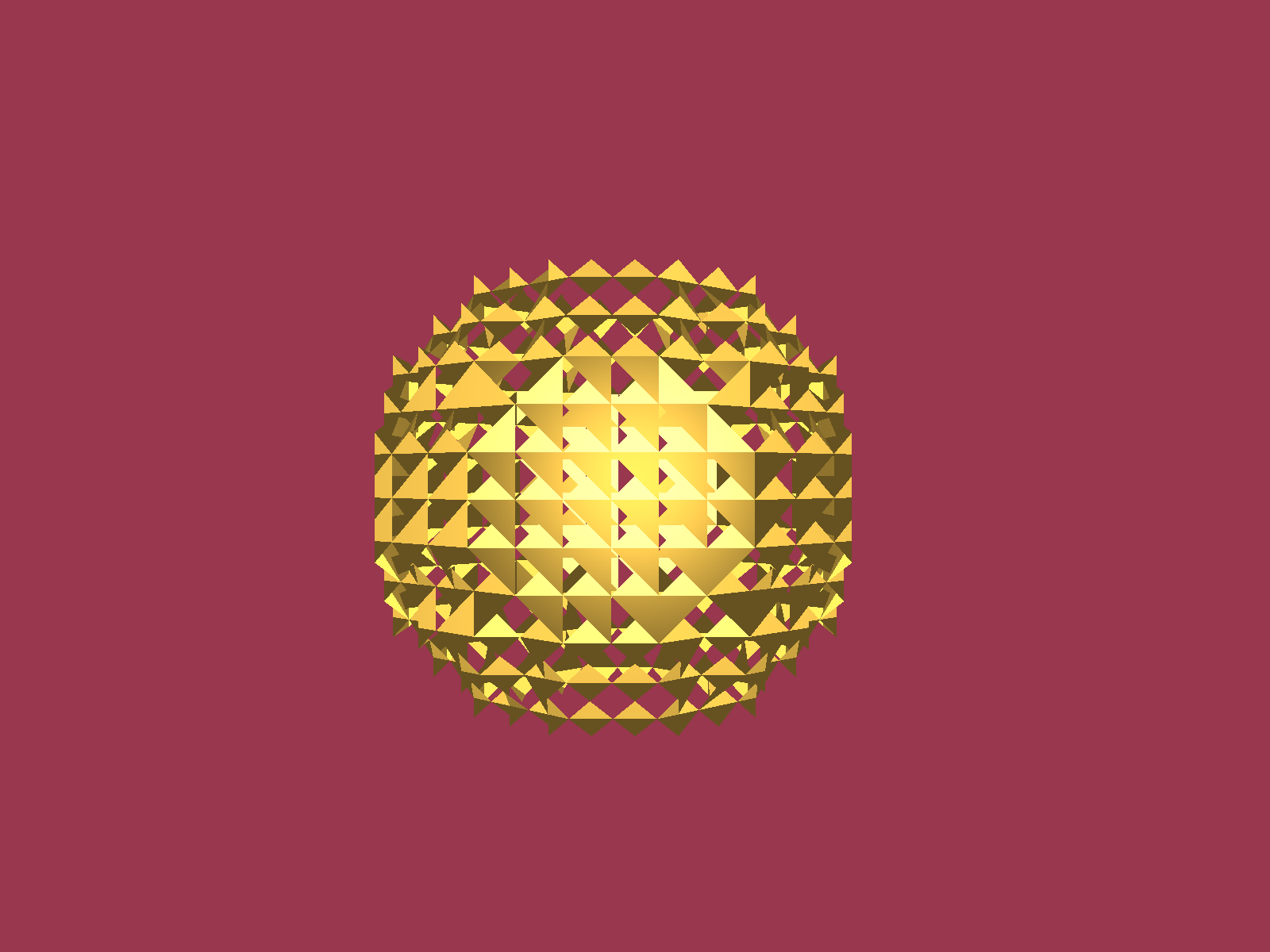Something isn't working.

I had clearly made a mistake somewhere along the way. At least I had got something (even if it was the wrong something) to draw. Anyone who has worked with OpenGL knows that sometimes you are not so lucky. One error hiding amongst all these moving parts may mean nothing renders at all.

Time to go bug hunting.

### Out of order

The indices looked out of order. The triangles were appearing in roughly the right place, but were being drawn incorrectly.

There were two probable causes. It could be that my linear index lookup code inside LatticeData3D was wrong. Either that, or something inside my modifications to the Polygonise function I had borrowed (and altered into polygoniseCell) was broken.

I quickly ruled out the index lookup code. Time to break down the polygoniseCell function in CubeLatticeScalarField3D. The main change I had made to this function when I integrated the original code into mine was removing the GRIDCELL struct. Rather than feed in the grid cell data with copies, I was looking up the data within from the 3D arrays directly. This was a little bit of effort for a pretty clear efficiency gain. Perhaps unnecessary, but I don't like passing things by copy when lookups or reference will work just fine.

Did I make a mistake in my implementation?

Oh, oops. I found a comment to myself left inside the following function:

std::array<size_t, 3>
CubeLatticeScalarField3D<sizeX, sizeY, sizeZ>::cellCornerIndexToIJKIndex(
size_t vertexIndex,
size_t i,
size_t j,
size_t k
);


The job of this function was to convert from a cell and vertex index into a location within the 3D array.

//TODO: Placeholder indices, double check correct order from the paper


I didn't fix the placeholder values. The code I was working with has an image showing the correct vertex index order required for the rest of the Polygonise function to work.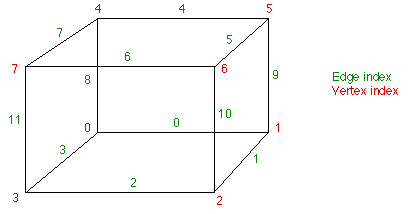Index order for the original Polygonise code from here.

I had left placeholder values in and forgotten to come back to it. I should've checked! At least I wrote myself a note. This is the sort of thing test driven development is designed to stop. I paid the price for working too fast.

I updated the index lookup order to match the correct order from the paper and hoped for the best.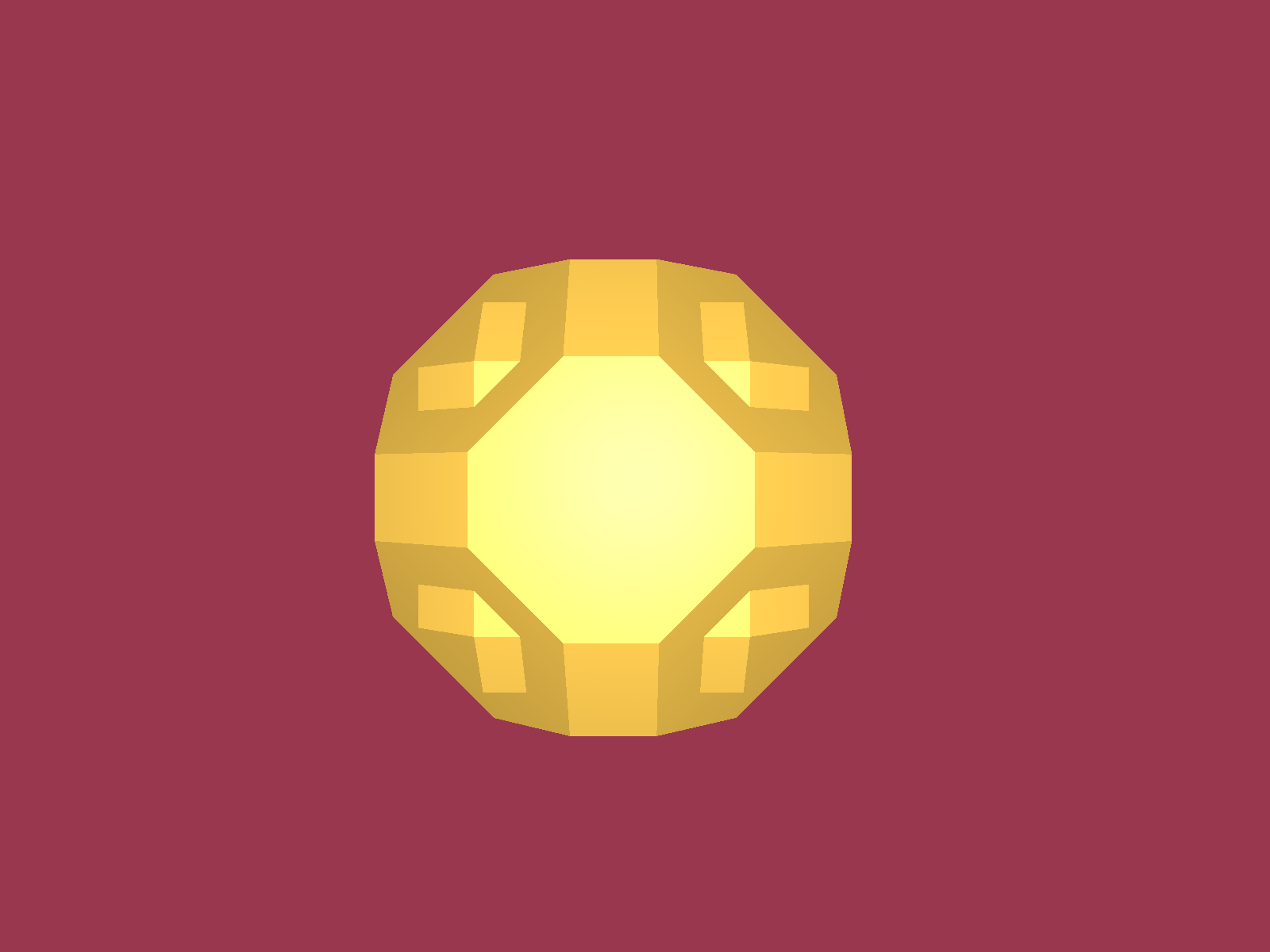Problem solved, note the facets (caused by the way the implicit surface was defined).

Now we were cooking, my marching cube code worked. Everything from this point was just icing on the cake.

## The camera

To show off the facets more clearly, I set the camera to rotate about the centre of the marching cube lattice region.

Windows, very handily, now includes screen capture tools. That saved me the effort. Writing screen dump tools is not so easy. To get reasonable speed, that would mean dealing with PBO's. No thanks, not for a small project anyway.

Rotating faceted sphere.

Even with the fixed frame loop, the rendering was smooth enough for the purposes of this article.

### Smoothing the facets with interpolation

To demonstrate the vertex interpolation feature designed into the marching cube code, I needed an implicit surface with smooth contour levels.

I altered the scalar field, $f(x,y,z)$, so that each value was defined by the function: $f(x,y,z) = \exp\left(-0.01\left(x^2+y^2+z^2\right)\right)$

Drawing the marching cubes should now result in a smooth sphere.

Rotating sphere with interpolated vertices.

The facets were still somewhat visible. This could be improved by computing more accurate vertex normals (rather than just using the surface normals). This can be done by building a proper index array for the vertices, then computing vertex normals using finite differences across adjacent triangle faces. Work for another day.

I was feeling unsatisfied. The sphere looked a little feature-less and kind of bland.

I wanted something that wasn't quite so symmetrical.

## Prettified outputs

I wanted to improve the visualisation a little before producing the end product. This would help remove the bland look of the sphere.

### Drawing the light source

The first thing was to add in a separate sphere (drawn using a polygon mesh, not with marching cubes) at the location of the light source. That way, it would be obvious where the light was coming from. The sphere code is from here. I added some modifications to make it easier to load the data into a VAO.

I also set this lighting sphere to rotate independently.

### Making a torus

I decided to convert my sphere into a torus. This would achieve my goal of drawing something a bit less symetrical.

I would do this by subtracting values away from the scalar field, dependent on the perpendicular distance from a line through the centre of the marching cube sphere I had already defined.

I used the following function $f(x,y,z) = \operatorname{max}\left[\exp\left(-0.01\left(x^2+y^2+z^2\right)\right) \exp\left(-0.3d(x,y,z)\right)\right]$ where $d(x,y,z)$ is the perpendicular distance of the point $(x,y,z)$ to the line intersection the points $(0,0,0)$ and $(1,0,2)$.

Code for the perpendicular distance can be found here or in the source code for this project.

More work than I intended, but at least it would look nice.

## Torus output

With all the code put together, it was finally time to check out the torus.

My first few attempts didn't really work. After messing around with the parameters controlling the exponential decay functions, I found something that looked like what I was after.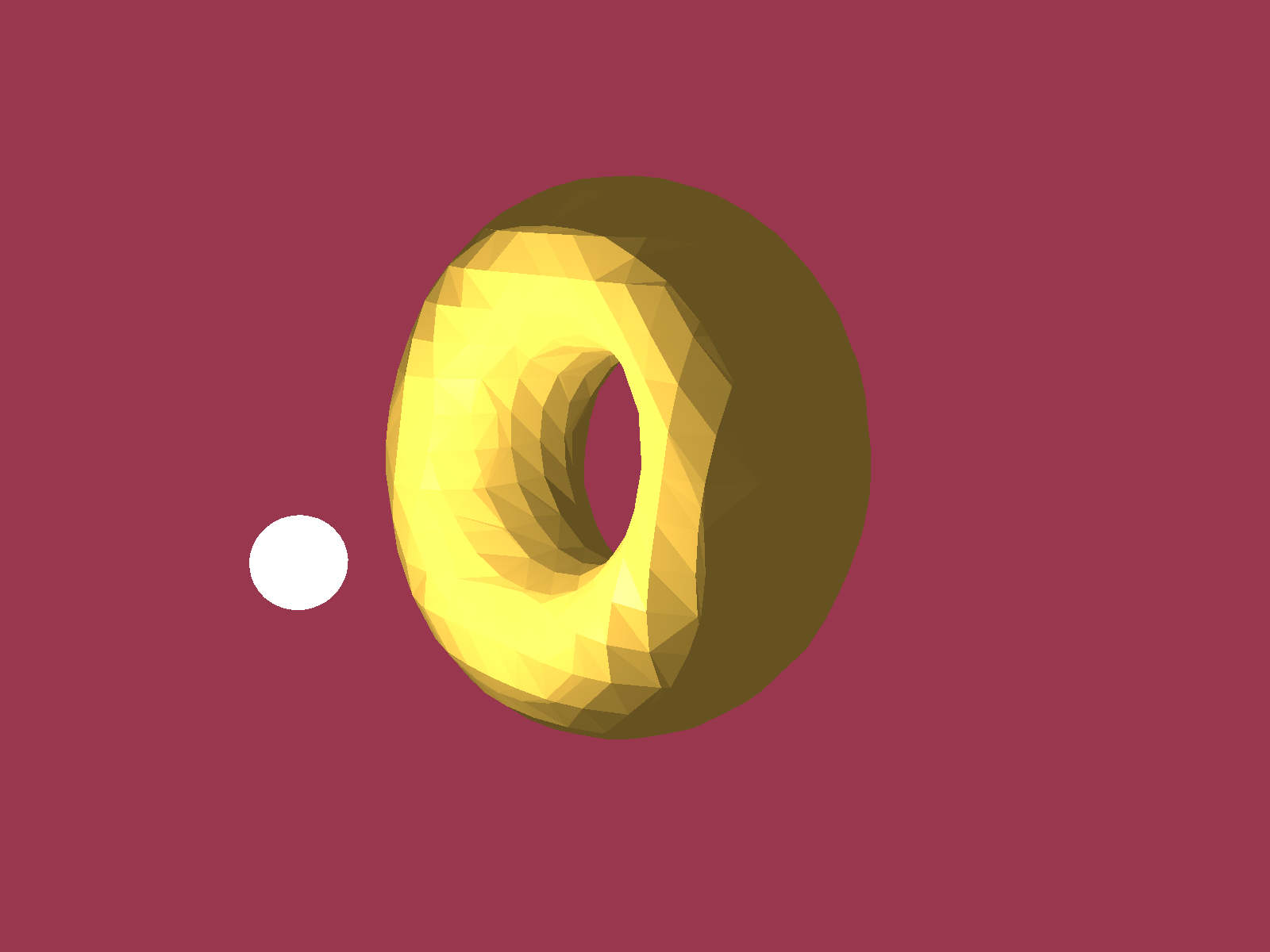Light near the torus.

I also spent some time altering the location of the light source and the camera, until I had something that I liked.

A light orbiting the torus.

The torus looked pretty good and, if I may say, perhaps even a little delicious. It could be the colour scheme, but I can't help but think about donuts.

## Conclusions

It was fun to write this code but overall I wasn't fully satisfied. I still wanted to test out dynamic loading and mesh reshaping (like in the Astroneer gif above). That extension to this code would be easy, but best left to another blog post.

Simulating spaces with depth and volume comes at additional computational cost. As cool as it is, sometimes it is fine to let the player imagine that the depth is there, rather than simulating it. The CPU cycles are only well spent if they have an impact on the player experience. Still, marching cubes are a nice trick to have up your sleeve.

I spent some more time playing with implicit surfaces. I like the video with two 'tunnels' at the start of the article. Download the source code and see what you can come up with. If you want some help or want to know more get in contact. The best place for quick response is on discord.

If you liked this article, please get in contact! You can send an email or chat in real time on discord.